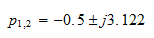# IC Electronic Filters Information

name="#top">

2. Types

## Introduction

Electronic filters are electrical circuits designed to remove, attenuate, or alter the characteristics of electrical signals. In particular these devices reduce the magnitude and the phase of unwanted signals with certain frequencies. A common example of an unwanted signal is noise, typically at 60 Hz. Filter operate by passing the system signal (voltage and noise) through a filter. If the filter is designed to suppress or attenuate the magnitude of the noise, its output will contain only (or mostly) the system signal. A typical radio receiver such as a car radio provides another good example. By tuning to a particular station the radio selects a particular signal while attenuating the signals of the other radio stations. This process is accomplished by a filter.

As an example, suppose a signal with frequency f1 is useful for a system, but an undesirable signal (noise) with frequency f2 has mixed with the useful signal, as shown below. Filters are used to suppress the second signal or reduce its amplitude. Part (a) of the figure shows the frequency response of the system, where the unwanted signal is prominent. After passing the signals through a filter designed to attenuate f2, the signal is reduced in amplitude with respect to f1.Filters are distinguished as analog or digital. Analog filters attenuate signals in analog systems, while digital filters attenuate digital signals in digital systems. This guide pertains to the design of analog filters.

The video below provides a comprehensive look at the material covered on this page, in lecture format.

name="operation">

## Operation

Suppose a medical technician is measuring a 5 Hz EKG sine wave signal from a patient. The measuring device, however, picks up instrument noise of 50 Hz, so that the actual measured signal is a mix of the noise and desired signal. The two signals must be separated to eliminate the noise. A low-pass filter similar in design to the above diagram is ideal in this situation. The compounded signal is passed through a filter with appropriate design values so that it almost completely attenuates the noise and extracts the EKG signal, as shown below.

The first and second plot of the figure show the original EKG desirable signal and the noise, respectively. The third plot shows the compounded signal, the input signal to the filter circuit. The last plot shows the extracted output signal from the filter. This output is the desired EKG signal. Notice that the peak-to-peak value of the output signal is smaller than the original desirable signal (the first plot in the figure). This is due to the fact that the filter did not completely remove the noise, but this output is reliable enough as to use it as the EKG signal. The output can be improved by selecting better filter parameters.name="types">

## Types

Filters are broadly classified according to the type of frequencies that the filter is able to suppress or attenuate. In this regard, there are four main categories:

• Low-pass filters attenuate or suppress signals with frequencies above a particular frequency called the cutoff or critical frequency. For example a low-pass filter (LPF) with a cutoff frequency of 40 Hz can eliminate noise with a frequency of 60 Hz.
• High-pass filters suppress or attenuate signals with frequencies lower than a particular frequency, also called the cutoff or critical frequency. For instance a high-pass filter (HPF) with a cutoff frequency of 100 Hz can be used to suppress the unwanted DC voltage in amplifier systems, if desired.
• Band-pass filters attenuate or suppress signals with frequencies outside a band of frequencies. They are commonly seen in TV or radio tuning circuits.
• Band-reject, or notch filters attenuate or suppress signals with a range of frequencies. For instance, a notch filter can be used to reject signals with frequencies between 50 Hz and 150 Hz.

name="description">

## Filter Descriptions

Filters are described using Laplace transforms and frequency-domain tools. In this way their amplitude and phase can be expressed as a function of frequency. The effect of filters on signals is also defined in terms of frequency-domain. A widely used mathematical tool to study filters is a graphical method called frequency response. This is a method used to study the dynamic behavior of systems by looking at the magnitude and phase of the output as a function of frequency. Typically, a filter system is excited with an input signal with a range of frequencies, and its output—magnitude and phase—are plotted as a function of frequency. The figure below shows the frequency response of a band-pass filter. The first graph shows the magnitude of the output as a function of frequency. In this case the magnitude is expressed in decibels (dB), and the frequency axis is a logarithmic axis due to the fact that normally the frequencies represent a large range. The bottom figure shows the phase (in degrees) as a function of frequency.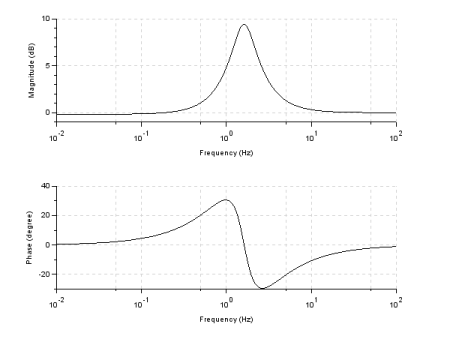name="ideal">

## Ideal Filters

To understand practical, real-world, filters, it is important to understand the behavior (frequency response) of ideal filters. These filters are impossible to build but are good teaching tools. An ideal filter frequency response consists of two or more sections of passband and stopband frequencies. The passband range comprises the frequencies where the response will show an amplitude with a fixed value or gain (normally 1). The stopband range of frequencies are the frequencies where the amplitude is zero. The frequencies at which the response changes from passband to stopband, or vice versa, are called critical frequencies or cutoff frequencies, the transition from stopband to passband and vice versa is instantaneous. The figure below shows the shape of the frequency response for the main four types of filters. Notice that for the bandpass filter (BPF) and the notch or bandstop filter (BS) there are two critical frequencies.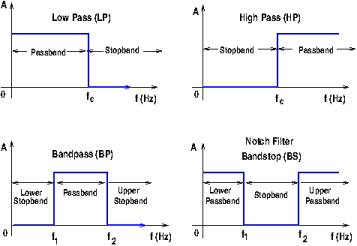The amplitude is normally the gain of the filter, or the ratio of output voltage and input voltage. In equation form the amplitude is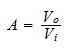If the filter circuit contains only passive elements (resistors, capacitors, inductors, etc.) the value of the amplitude for an ideal filter is 1.0, but if the filter is built with active components (transistors, op-amps, etc.) the value of the amplitude (the gain) is different from 1.0. (For more information about passive filters, visit the Passive Filters Specification Guide.)

It is convenient to express the amplitude of the response in decibels (dB). The following equation converts the amplitude from raw numbers (ratio) to decibels.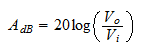For passive filters the constant amplitude expressed in dB is zero, or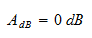name="practical">

## Practical Filters

It is practically impossible to instantaneously transition from stopband to passband or vice versa, as in the above discussion about ideal filters. Instead of the transition represented by ideal filters, a closer behavior to that of ideal-real filters contains a transition region between the passband and the stopband. There is always a transition range between these two regions, so practical-ideal or real-ideal filters have a frequency response shown in the figure below.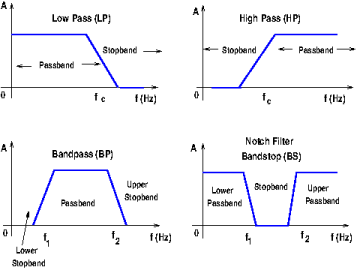However, this figure still does not represent a practical, real-world filter. The response amplitude passband is typically not a straight, flat horizontal line: it may have attenuation ripples. The transition is not a straight line either. There are oscillations produced by real components that must be taken into account. The stopband may have ripples as well. A more accurate depiction of the frequency response is shown in the low-pass filter response graph below.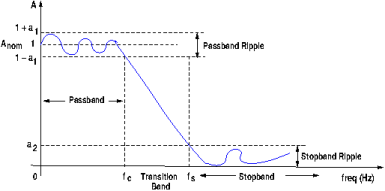As shown in the above graph, there are several parameters in a practical filter:

• Passband: The range of frequencies where the output has a gain.
• Stopband: The range of frequencies where the output is zero or very small.
• Passband ripple: The variations or oscillations in the bandpass, also called error band. In a typical filter, these oscillations occur around the nominal value of 1.0, or at 0 dB, if the amplitude is expresses in decibels. The ripple value is 2a1, where a1 is a parameter dependent on the circuit components.
• Stopband ripple: This represents the variations in the stopband region. The ripple is equal to the parameter a2, which is determined by the values of the circuit components.
• Critical frequency, fc: This is the frequency at which the response leaves the passband ripple. For certain type of filters (Butterworth filters, for instance), at this frequency the amplitude of the response is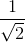of the nominal amplitude. If the nominal amplitude is Anom the value of the amplitude at fc can be determined by using the following equation: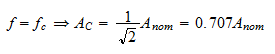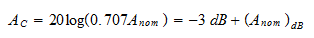The nominal value in this figure is 1.0, but it can be larger, particularly in active filters.

• Stopband frequency, fs: This is the frequency at which the maximum stopband ripple (a2) occurs.
• Transition band: This represents the range (fs - fc) of frequencies between the critical and cutoff frequencies. The slope or steepness of the transition region is related to the number of poles in the transfer function of the response, also known as the order of the filter. A pole is a root of the denominator of the transfer function. For a standard Butterworth filter every pole adds  -20  dB/decade or -6 dB/octave to the slope of the response. The slope of the line is called the roll-off of the transition. Also, a pole represents one RC stage in the circuit, as shown below. A filter that has only one RC network it is called a single-pole or order-one filter; one with two RC circuits is a 2-pole or second order filter, and so on.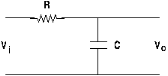name="response">

## Response Characteristics

The frequency response of any filter can be designed by properly selecting the circuit components. Filter characteristics are defined by the shape of the frequency response curve.

The most important response shapes are named after a researcher who studied the particular filters. These include Butterworth, Chebyshev (types I and II) , elliptic (or Cauer), and Bessel types. Each one of these filter types has a particular advantage in certain applications.  The characteristics of four low-pass filters, each one of three-poles and cutoff frequency of 10, is shown below.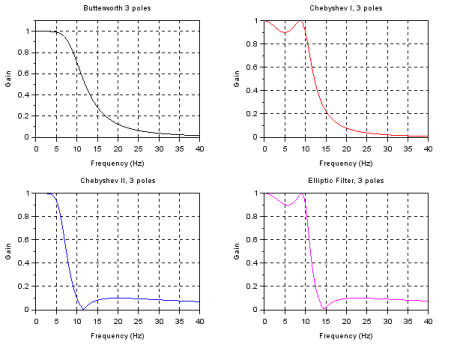name="transfer">

## Transfer Function

Filters operate on the frequency of signals. Therefore the most powerful tools to describe the behavior of filters are analytical and graphical descriptions using the frequency domain. Thus, frequency domain equations and curves of gain vs. frequency and phase vs. frequency are commonly used. To study the frequency domain of networks their mathematical description in terms of the transfer function of the system is required. For a general system, as depicted below, the voltage transfer function is the ratio of the Laplace transforms of the output and the input signals.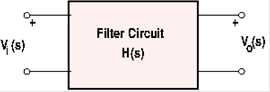For the system shown above the transfer function is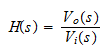where Vi(s)  and Vo(s) are the input and output voltages and s is the complex Laplace transform variable. In terms of the frequency of the system the Laplace variable is given by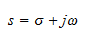where ω=2πf is the angular frequency in radians per second and σ is the Neper frequency in nepers per second. For casual systems with output dependent on present and past inputs only, σ=0. Therefore s= for the filter's characteristics.

Most electrical systems are frequency-dependent because the impedances of capacitors and inductors are frequency dependent, given byor, for a capacitor with capacitance C (farads)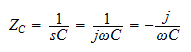and for an inductor with inductance L (Henry)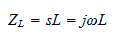Two important sub-equations are derived from the transfer function. The amplitude response represents the magnitude of the complex transfer function as a function of frequency. This describes the effect of the filter on the amplitudes of input signals at various frequencies. The second sub-equation is the phase response of the filter. This equation tells us the amount of phase shift introduced in sinusoidal input signals as a function of frequency. A phase shift of a signal represents a change in time of the output with respect to the input.

Replacing s with  in the transfer function equation determines the amplitude and phase response. The amplitude response is the magnitude and is given by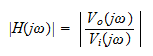and the phase response is written as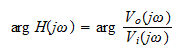The transfer function of any circuit can be found by using standard network techniques such as Ohm's law, Kirchoff's laws, and superposition. By using complex impedances for capacitors and inductors, the standard form of the transfer function is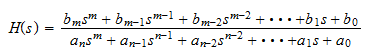H(s) is a rational function in s and with real coefficients. It is convenient to factor the numerator and denominator polynomials and to write the transfer function accordingly: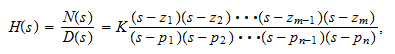where N(s) and D(s) are the real coefficient numerator and denominator, respectively and K=bm / an. In this equation the zeros (zi) are the roots of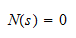and the poles pi are the roots of the equation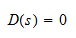The degree of the numerator is m and the degree of the denominator is n.  This equation is expressed such that when s=zi,  for i=1...m the numerator becomes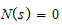and the transfer function becomes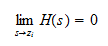Similarly, when s=pi, for i=1...m the denominator becomes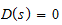and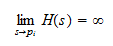Notice that the coefficients of the numerator and denominator polynomials are real numbers. Therefore the roots must be either real numbers or appear in pairs of complex conjugate numbers. The numerator may be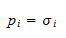or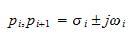Likewise, for the denominator.

The degree of a filter's denominator is the order of the filter, and the roots of the denominator are the poles of the filter. The roots of the numerators are called the zeros of the filter. Each pole provides -20 dB/decade or -6 dB/octave.

So if a Butterworth filter has 3 poles, and no zeros the slope of the transition band is -60 dB/decade.

### A Practical Example

As an example consider the second order low-pass filter with implementation as shown in the following figure.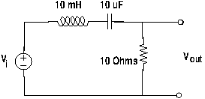The transfer function can be found by applying the voltage divider rule across the resistance: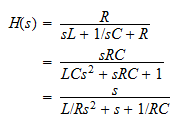Substituting the values of the circuit, the equation becomes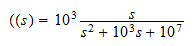Factorizing the denominator, it becomes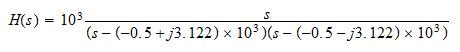Therefore the filter has a zero at that origin (s=0) and two complex conjugate poles at